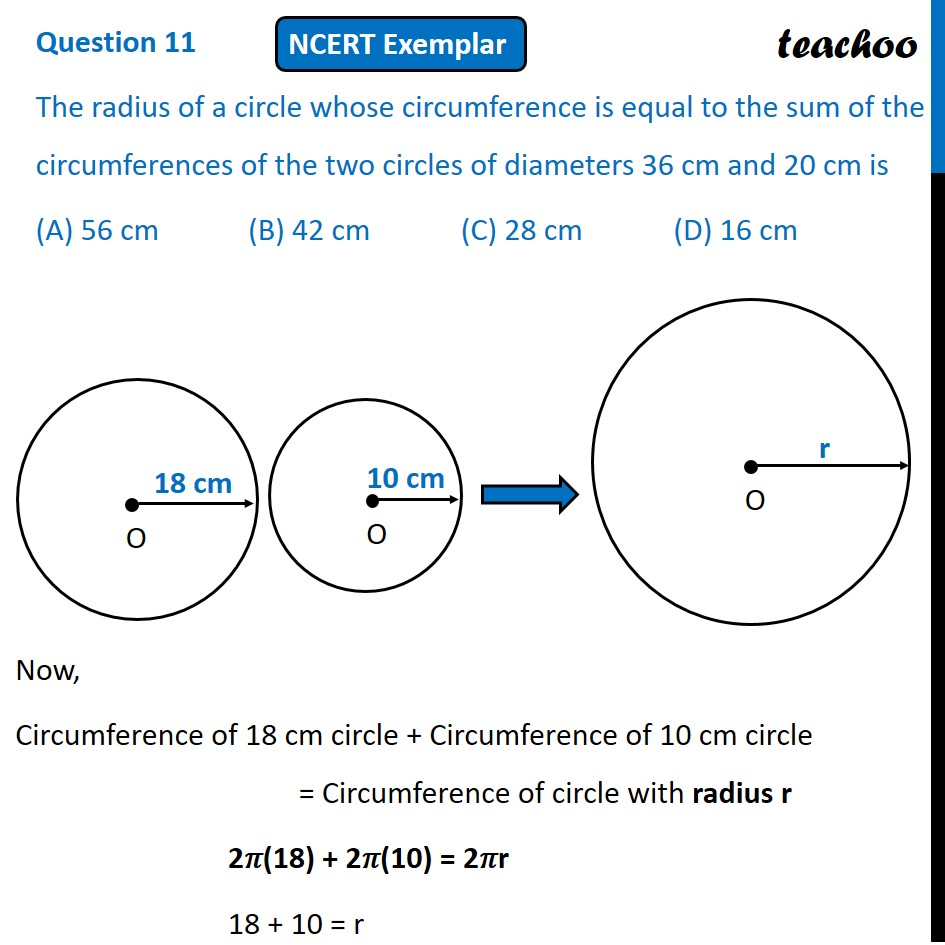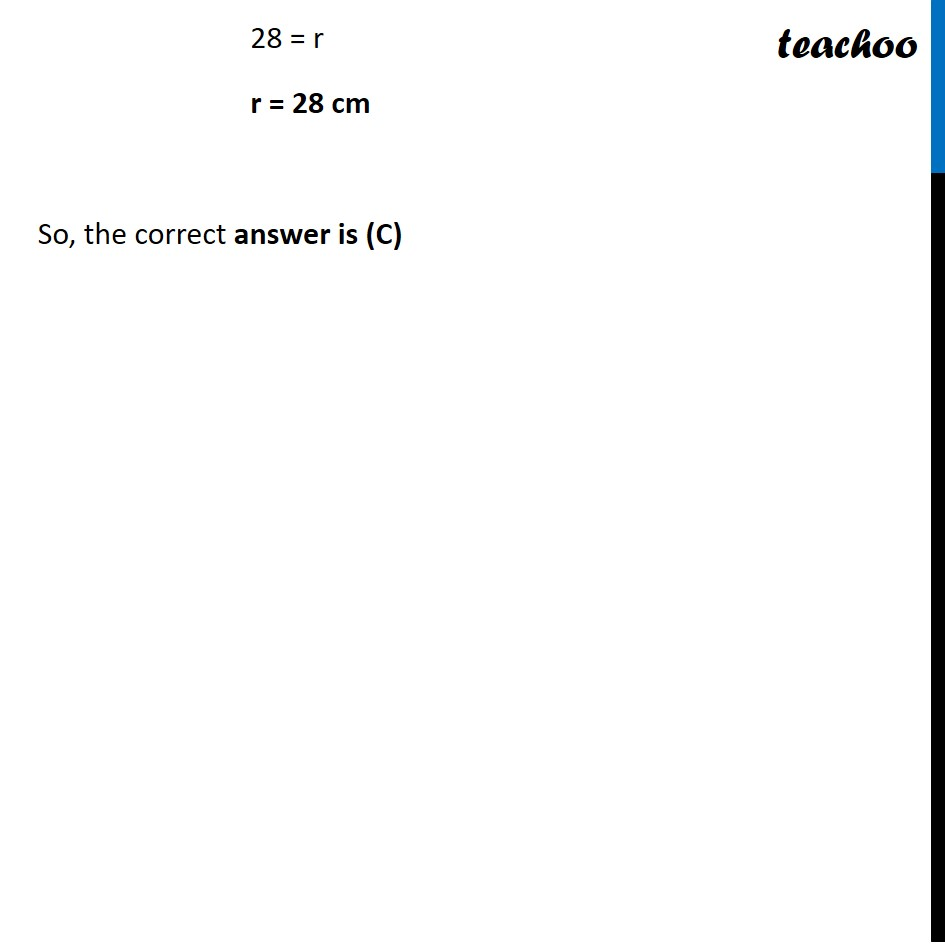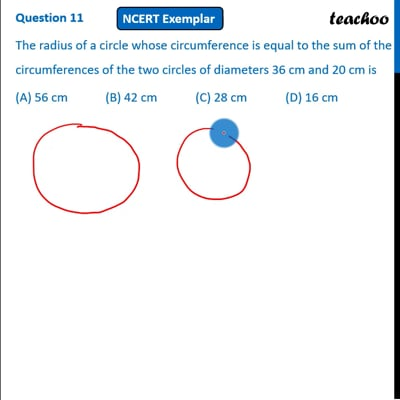NCERT Exemplar - MCQ

Chapter 12 Class 10 Areas related to Circles
Serial order wise

## (A) 56 cm   (B) 42 cm   (C) 28 cm   (D) 16 cmThis video is only available for Teachoo black users

Maths Crash Course - Live lectures + all videos + Real time Doubt solving!

### Transcript

Question 11 The radius of a circle whose circumference is equal to the sum of the circumferences of the two circles of diameters 36 cm and 20 cm is (A) 56 cm (B) 42 cm (C) 28 cm (D) 16 cm Now, Circumference of 18 cm circle + Circumference of 10 cm circle = Circumference of circle with radius r 2𝜋(18) + 2𝜋(10) = 2𝜋r 18 + 10 = r 28 = r r = 28 cm So, the correct answer is (C)